Open in App
Not now

# Invertible Functions

• Difficulty Level : Expert
• Last Updated : 07 Dec, 2021

As the name suggests Invertible means inverse“, Invertible function means the inverse of the function. Inverse functions, in the most general sense, are functions that “reverse” each other. For example, if f takes a to b, then the inverse, f-1, must take b to a.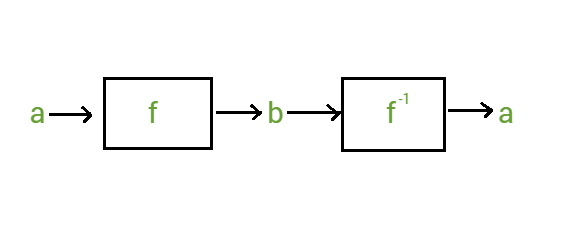The inverse of a function is denoted by f-1

In other words, we can define as, If f is a function the set of ordered pairs obtained by interchanging the first and second coordinates of each ordered pair in f is called the inverse of f. Let’s understand this with the help of an example.

Example:

function g = {(0, 1), (1, 2), (2,1)}, here we have to find the g-1

As we know that g-1 is formed by interchanging X and Y co-ordinates.

g = {(0, 1), (1, 2), (2, 1)}  -> interchange X and Y, we get

g-1 = {(1, 0), (2, 1), (1, 2)}

So this is the inverse of function g.

### Graph of Inverse Function

We can check for the function is invertible or not by plotting on the graph. We can plot the graph by using the given function and check for invertibility of that function, whether the function is invertible or not. Let’s plot the graph for the function and check whether it is invertible or not for f(x) = 3x + 6. This function has intercept 6 and slopes 3. Let’s plot the graph for this function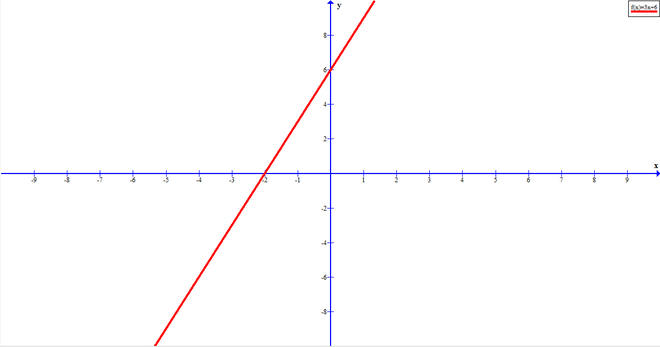Example:

Let’s find out the inverse of the given function.

f(x) = 3x + 6

Interchange x with y
x = 3y + 6
x – 6 = 3y

y = (x – 6) / 3

y = (1 / 3)x / 3

f-1(x) = (1 / 3) x / 3

Now let’s plot the graph for f-1(x). The inverse of a function having intercept and slope 3 and 1 / 3 respectively.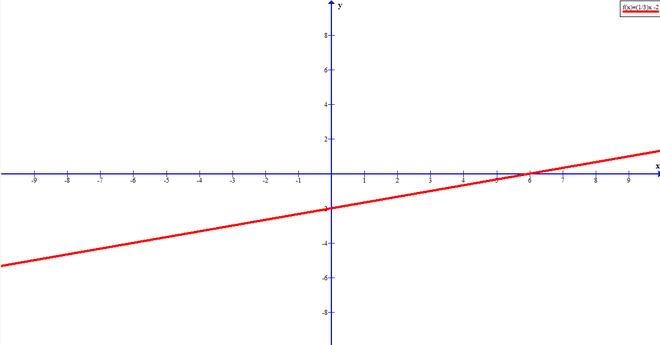A function and its inverse will be symmetric around the line y = x. Then the function is said to be invertible. So let’s draw the line between both function and inverse of the function and check whether it separated symmetrically or not.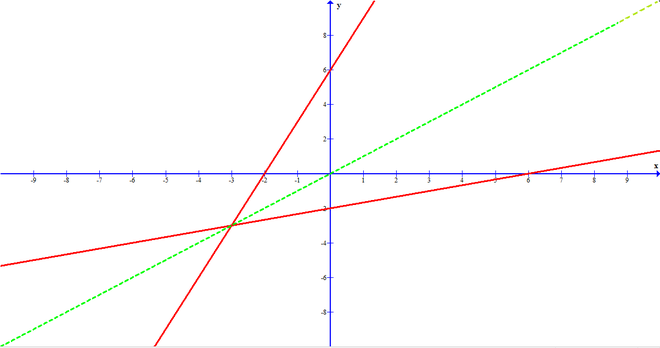After drawing the straight line y = x, we observe that the straight line intersects the line of both of the functions symmetrically. So, the function f(x) is an invertible function and in this way, we can plot the graph for an inverse function and check the invertibility.

### Conditions for the Function to Be Invertible

Condition: To prove the function to be invertible, we need to prove that, the function is both One to One and Onto, i.e, Bijective.

#### Explanation

We can say the function is One to One when every element of the domain has a single image with codomain after mapping. We can say the function is Onto when the Range of the function should be equal to the codomain. When we prove that the given function is both One to One and Onto then we can say that the given function is invertible. Let’s see some examples to understand the condition properly.

Example 1: Let A : R – {3} and B : R – {1}. Consider the function f : A -> B defined by f(x) = (x – 2) / (x – 3). Show that function f(x) is invertible and hence find f-1.

Solution:

To show the function is invertible, we have to verify the condition of the function to be invertible as we discuss above. To show that the function is invertible we have to check first that the function is One to One or not so let’s check.

Let x, y ∈ A such that f(x) = f(y)

=> (x – 2) / (x – 3) = (y – 2) / (y – 3)

=> (x – 2) (y – 3) = (x – 3) (y – 2)

=> xy – 3y – 2y + 6 = xy – 2x – 3y + 6

=> -3x + 2y + 6 = xy – 2x – 3y + 6

=> -3x + 2x = -3 + 2y

=> -x = -y
=> x = y

Since f(x) = f(y) => x = y, ∀x, y ∈ A, so function is One to One.

We have proved the function to be One to One. Now let’s check for Onto. To show that f(x) is onto, we show that range of f(x) = its codomain.

Let y = (x – 2) / (x – 3)

Put f(x) = y.

=> xy – 3y = x – 2

=> xy – x = 3y – 2

=> x(y – 1) = 3y – 2

=> x = (3y – 2) / (y -1)    —-(1)

Since x ∈  R – {3}, ∀y R – {1}, so range of f is given as = R – {1}. Also codomain of f = R – {1}.

Therefore, Range = Codomain => f is Onto function

As both conditions are satisfied function is both One to One and Onto, Hence function f(x) is Invertible. Now as the question asked after proving function Invertible we have to find f-1

from eq(1) we get,

f-1(y) = (3y – 2) / (y – 1)

=> f-1(x) = (3x – 2) / (y – 1)

Example 2: Show that f: R – {0} -> R – {0} given by f(x) = 3 / x is invertible.

Solution:

To show the function f(x) = 3 / x is invertible.

We have to check first whether the function is One to One or not.

Let x1, x2 ∈ R – {0}, such that  f(x1) = f(x2). Then,

f(x1) = f(x2)

=> 3 / x1 = 3 / x2
=> x1 = x2

Thus, f(x1) = f(x2

=> x1 =x2 ∀x, y ∈  R – {0}

So, function f is One to One.

We have proved that the function is One to One, now let’s check whether the function is Onto or not.

Let y be an arbitrary element of  R – {0}.

Then for y in the codomain  R – {0},

there exist its pre-image in the domain  R – {0}. So f is Onto.

Since we proved the function both One to One and Onto, the function is Invertible.

Example 3: Consider f: R+ -> [4, ∞] given by f(x) = x2 + 4. Show that f is invertible, where R+ is the set of all non-negative real numbers.

Solution:

To show that the function is invertible or not we have to prove that the function is both One to One and Onto i.e, Bijective

Let’s check for One to One.

Here function f: R+ -> [4, infinity)

Given by f(x) = x2 + 4,

Let x, y ∈ R+, such that f(x) = f(y)

=> x2 + 4  = y2 + 4

=> x2 = y2

=> x = y [Since we have to take only +ve sign as x, y ∈ R+]

Therefore, f is One to One function.

Now, we have to check for Onto.

For y ∈ [4, infinity), let y = x2 + 4

=> x2 = y – 4 ≥ 0

=> x = √(y – 4) ≥ 0 [we take only +ve sign, as x ∈ R+]

Therefore, for any y ∈ R+ (codomain), there exists

x = √(y – 4) R+ (domain) such that

f(x) = f(√(y-4)) = (√(y – 4))2 + 4 = y – 4 + 4 = y

Therefore, f is Onto function.

Since function f(x) is both One to One and Onto, function f(x) is Invertible.

### Determining If a Function is Invertible

As we had discussed above the conditions for the function to be invertible, the same conditions we will check to determine that the function is invertible or not. So let’s take some of the problems to understand properly how can we determine that the function is invertible or not.

Example 1: If f is an invertible function, defined as f(x) = (3x -4) / 5 , then write f-1(x).

Solution:

In the question given that f(x) = (3x – 4) / 5 is an invertible and we have to find the inverse of x. So, firstly we have to convert the equation in the terms of x. In the below figure, the last line we have found out the inverse of x and y. So, this is our required answer.

Given, f(x) (3x – 4) / 5 is an invertible function.

Let, y = (3x – 5) / 5
5y = 3x – 4
3x = 5y + 4
x = (5y – 4) / 3

Therefore, f-1(y) = (5y – 4) / 3 or f-1(x) = (5x – 4) / 3

Example 2: f : R -> R defined by f(x) = 2x -1, find f-1(x)?

Solution:

As we done in the above question, the same we have to do in this question too. In the question we know that the function f(x) = 2x – 1 is invertible. Let us have y = 2x – 1, then to find its inverse only we have to interchange the variables.

Given,

f(x) = 2x -1 = y is an invertible function.

Let, y = 2x – 1
Inverse: x = 2y – 1
therefore, f-1(x) = (x + 1) / 2

Example 3: Show that the function f: R -> R, defined as f(x) = 4x – 7 is invertible of not, also find f-1.

Solution:

In the question, given the f: R -> R function f(x) = 4x – 7. We have to check if the function is invertible or not. So, to check whether the function is invertible or not, we have to follow the condition in the above article we have discussed the condition for the function to be invertible. So as we learned from the above conditions that if our function is both One to One and Onto then the function is invertible and if it is not, then our function is not invertible. So, let’s solve the problem firstly we are checking in the below figure that the function is One-One or not.

One-One function means that every element of the domain have only one image in its codomain. So we had a check for One-One in the below figure and we found that our function is One-One. Now, the next step we have to take is, check whether the function is Onto or not. The function is Onto only when the Codomain of the function is equal to the Range of the function means all the elements in the codomain should be mapped with one element of the domain. So, we had checked the function is Onto or not in the below figure and we had found that our function is Onto. So, the condition of the function to be invertible is satisfied means our function is both One-One Onto. Hence we can prove that our function is invertible.

Given, f : R -> R such that f(x) = 4x – 7

For one to one:

Let x1 and x2 be any elements of R such that f(x1) = f(x2), Then

f(x1) = f(x2)
4x1 – 7 = 4x2 – 7
4x1 = 4x2
x1 = x2
So, f is one to one

For onto:

Let y = f(x), y belongs to R. Then,
y = 4x – 7
x = (y+7) / 4

From above it is seen that for every value of y, there exist it’s pre-image x.

So, f is onto

Thus, f is being One to One Onto, it is invertible.

### Inverse Trigonometric Functions

Inverse functions are of many types such as Inverse Trigonometric Function, inverse log functions, inverse rational functions, inverse rational functions, etc. In the below table there is the list of Inverse Trigonometric Functions with their Domain and Range.

### Finding Inverse Function Using Algebra

Example 1: Find the inverse of the function f(x) = (x + 1) / (2x – 1), where x ≠ 1 / 2

Solution:

For finding the inverse function we have to apply very simple process, we  just put the function in equals to y.

(x+1) / (2x-1) = y

x +1 = 2xy – y

x- 2xy = -y – 1

x(1- 2y) = -y – 1

x = (-y – 1) / (1 – 2y)

By taking negative sign common, we can write ,

x = (1 + y) / (2y – 1)

f-1(x) = (1 + x / (2x – 1)

This is the required inverse of the function.

Example 2: Solve: f(x) = 2x  /  (x -1)

Solution:

As we done above, put the function equal to y, we get

2x / (x – 1) = y

2x = xy – y

2x – xy = -y

Taking x common from the left hand side

x (2 – y) = -y

x = -y / (2 – y)

Taking y common from the denominator we get,

x = y / (y – 2)

f-1(x) = x / (x – 2)

This is required inverse of the function.

Example 3: Find the inverse for the function f(x) = 2x2 – 7x +  8

Solution:

We follow the same procedure for solving this problem too,

put the function equal to y, we get

2x2 – 7x +  8 = y

Taking 2 common from left hand side

2 [ x2 – (7 / 2)x +  4] = y

To make the perfect square of (x – y)2

we have to divide and multiply by 2 with second term of the expression.

Adding and subtracting 49 / 16 after second term of the expression.

We get,

2[ x2 – 2. (7 / 2*2). x + 49 / 16 – 49 / 16 +4] = y

See carefully the underlined portion, it is the formula (x – y)2 = x2 – 2xy + y2

We can write this,

2(x – (7 / 4))2 – 49 / 8 + 8 = y

2(x – (7 / 4))2 = y – (15 / 8)

(x – (7 / 4))2 = (y / 2) – (15 / 8)

Now, remove square root,

x – (7 / 4) = square-root((y / 2) – (15 / 32))

x = (7 / 4) + square-root((y / 2) – (15 / 32))

f-1(x) = (7 / 4) + square-root((x / 2) – (15 / 32))

This is the required inverse of the function.

### Restricting Domains of Functions to Make them Invertible

As the above heading suggests, that to make the function not invertible function invertible we have to restrict or set the domain at which our function should become an invertible function. We know that the function is something that takes a set of number, and take each of those numbers and map them to another set of numbers. So if we start with a set of numbers.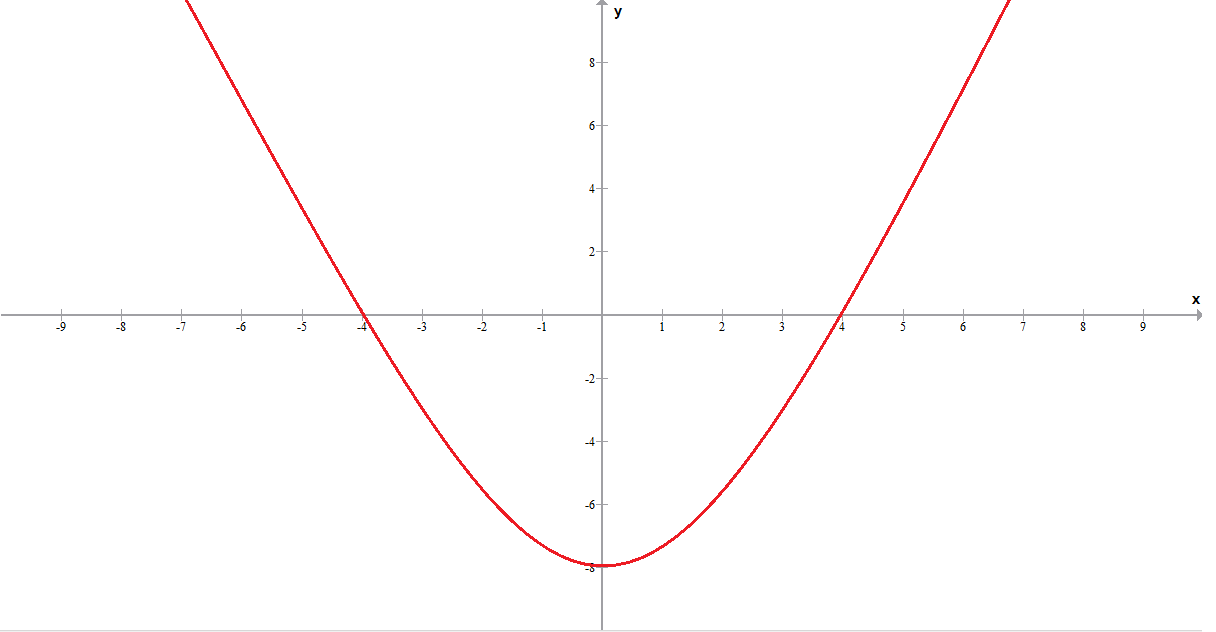The above table shows that we are trying different values in the domain and by seeing the graph we took the idea of the f(x) value. When x = 0 then what our graph tells us that the value of f(x) is -8, in the same way for 2 and -2 we get -6 and -6 respectively. As we see in the above table on giving 2 and -2 we have the output -6 it is ok for the function, but it should not be longer invertible function. So, in the graph the function is defined is not invertible, why it should not be invertible?, because two of the values of x mapping the single value of f(x) as we saw in the above table. In the order the function to be invertible, you should find a function that maps the other way means you can find the inverse of that function, so let’s see

So if we find the inverse, and we give -8 the inverse is 0 it should be ok, but when we give -6 we find something interesting we are getting 2 or -2, it means that this function is no longer to be invertible, demonstrated in the below graph.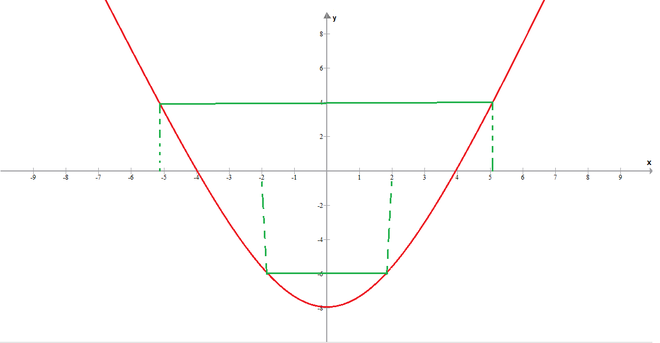In the same way, if we check for 4 we are getting two values of x as shown in the above graph. Now, we have to restrict the domain so how that our function should become invertible. So, we can restrict the domain in two ways

• (0, ∞)
• (-∞, 0)

Let’s try first approach, if we restrict domain from 0 to infinity then we have the graph like this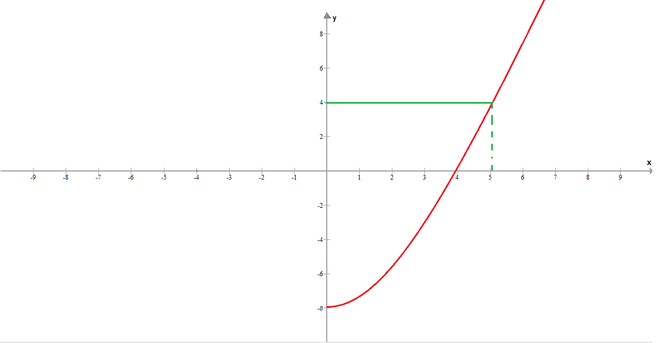We have this graph and now when we check the graph for any value of y we are getting one value of x, in the same way, if we check for any positive integer of y we are getting only one value of x. Now, let’s try our second approach, in which we are restricting the domain from -infinity to 0. If we plot the graph our graph looks like this.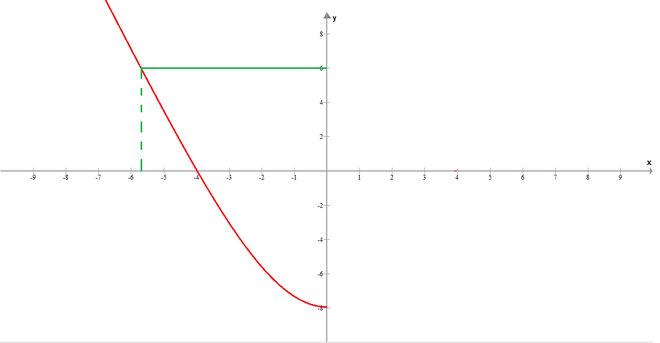In this graph we are checking for y = 6 we are getting a single value of x. Now if we check for any value of y we are getting a single value of x. So in both of our approaches, our graph is giving a single value, which makes it invertible. So, our restricted domain to make the function invertible are

• (0, ∞)
• (-∞, 0)

My Personal Notes arrow_drop_up